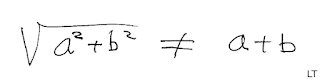## Thursday, January 5, 2017

### CalculatorsKids are novices, not little mathematicians! They count on their fingers until they memorize single-digit number facts and learn the standard algorithms for calculating.

1. Calculators should not be used as a substitute for weak arithmetic skills.
2. The standard algorithms should be taught first as the primary method for calculating.

Kids are novices, not little mathematicians.
A mathematician, who apparently knows little about how children learn math or the cognitive science of learning, advocates the same old [failed] NCTM reform math doctrine. He writes, "Today's citizen no longer needs to learn or practice the use of routine procedures to a level of fluency because devices do procedural math for us much faster and without virtually no chance of error. What we need is an understanding of mathematical concepts and methods" to make proper use of the calculating tools "to solve problems in our work and our lives." Really? I diverge. The mathematician, whose name I will not mention, has lost sight of the fact that you have to be able to do a procedure to understand it.But how would you know that the square root of the sum of two squares is not equal to a + b unless you could do the procedure and have some understanding of the idea? For example, if a = 5 and b = 6, then the square root of (25 + 36) or √61 would be approximately 7.81 to two decimal places. The result would not be a + b or 5 + 6 = 11. Likewise, if you compute the hypotenuse of a right triangle with side lengths of 3 and 4, it would not be 3 + 4 = 7.  The square root of the sum of 9 and 16 or √25 is 5, according to the Pythagorean Theorem. The hypotenuse is 5, not 7. Incidentally, the square of the (square root of the sum of two squares ) is a^2 + b^2.

To learn factual knowledge or standard procedural knowledge means to be able to recall it and do it (apply it). In short, children will not gain much number sense without learning and doing a lot of basic arithmetic starting in 1st grade. Memorization and "drill to improve skill" are both essential to cement fundamentals in long-term memory for use in standard algorithms and problem-solving.

The anti-knowledge movement has been very active in U.S. education. Apparently, students don't need to learn factual knowledge and standard procedural knowledge in math to learn math. All students need is critical thinking. No need to memorize the single-digit number facts or learn the whole number standard algorithms, fractions, long division, solving equations, or word problems. Instead, students should learn calculator skills and be familiar with arithmetic even though they can't perform it. Really?

"Mathematics builds new ideas on old ones. ... so, it is [hierarchical and ] logical. Everything fits together," writes Ian Stewart (Letters to a Young Mathematician, 2006). Like most mathematicians, Stewart thinks beginners must learn arithmetic basics first. Contrary to Common Core reform math, you don't ask novices to attend to precision, use appropriate tools strategically, construct viable arguments or argue from evidence, show many different ways to prove an answer, and a bunch of other extras. Arithmetic is not a matter of opinion. Parents will be happy to know that 3 + 5 is still 8 and should be memorized and that the standard algorithm is the primary method for calculating.

Stewart also points out, "One of the biggest differences between school math and university math is proof. At school we learn how to solve equations or find the area of a triangle; at university, we learn why those methods work and prove that they do." While mathematicians are obsessed with proof, beginners should spend most of their time on the how rather than the why. Children should not be asked to prove their answer correct by making a drawing, using several different algorithms, or writing a paragraph. The conventional method of showing one's work is sufficient.

Calculators are "absolutely unnecessary."

W. Stephen Wilson, a math and education professor at Johns Hopkins University, points out,  I have not yet encountered a mathematics concept that required technology to either teach it or assess it. The concepts and skills we teach are so fundamental that technology is not needed to either elucidate them or enhance them. There might be teachers who can figure out a way to enhance learning with the use of technology, but it’s absolutely unnecessary." And, it eats up instructional time. For example, a popular Algebra-2 textbook includes 4 pages of using the TI-84 graphing calculator for each of the 14 chapters.

William Pang writes in The Atlantic*, "If a student can't master long division, how can they grasp derivatives and integrals?" Not all students will take calculus, of course, but we must prepare kids properly and give them a good grasp of math facts, concepts, and standard procedures for proficiency to prepare for university math courses and other areas of study that require a solid math background (e.g., science, economics, engineering, business, etc.).

Often, high school math homework problems require the use of graphing calculators. In contrast, nearly half of the university math instructors don't allow calculators on Calculus-1 exams, based on a 2010 survey by the Mathematical Association of America, says Pang.

Unfortunately, calculators are used in prealgebra and algebra textbooks, the GED and SAT, AP courses, etc. Dependence on calculators has concealed weak arithmetic skills.

*The Atlantic: "The Common High-School Tool That's Banned in College," by William Pang, December 22, 2016

Note: In a 1st-grade Russian classroom, there was a banner above the blackboard:

2 + 3 = 5 and 5 - 3 = 2     3 ● 4 = 12 and 12 : 4 = 3
The symbol ●  means multiplication. The symbol : means division.